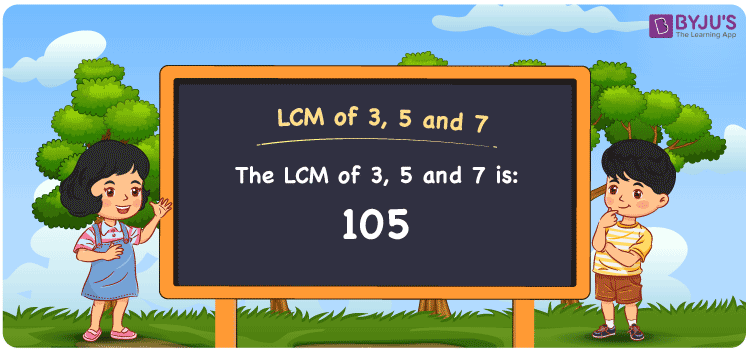# LCM of 3 5 and 7

LCM of 3, 5 and 7 is 105. LCM is the method to find the smallest possible multiple of two or more numbers. Professional teachers prepared the article LCM of Two Numbers with the aim to offer comprehensive learning and build better maths skills among students. This article explains the concept in a detailed manner to make learning much more comfortable for students. In this article, let us learn in detail how to find the least common multiple of 3, 5 and 7 using prime factorisation, division method and a list of multiples in a clear format.

## What is LCM of 3, 5 and 7?

The Least Common Multiple of 3, 5 and 7 is 105.## How to Find LCM of 3, 5 and 7?

LCM of 3, 5 and 7 can be determined using three methods:

• Prime Factorisation
• Division method
• Listing the Multiples

### LCM of 3, 5 and 7 Using Prime Factorisation Method

In this method, the given natural numbers are written as the product of prime factors. The least common multiple will be the product of all prime factors with the highest degree. Hence, the numbers 3, 5 and 7 can be expressed as;

3 = 3

5 = 5

7 = 7

LCM (3, 5, 7) = 3 × 5 × 7 = 105

### LCM of 3, 5 and 7 Using Division Method

In the division method, to calculate the least common multiple of 3, 5 and 7, we divide the numbers 3, 5 and 7 by their prime factors until we get the result as one in the complete row. The product of these divisors depicts the least common multiple of 3, 5 and 7.

 3 3 5 7 5 1 5 7 7 1 1 7 x 1 1 1

No further division can be done.

Hence, LCM (3, 5, 7) = 3 × 5 × 7 = 105

### LCM of 3, 5 and 7 Using Listing the Multiples

In this method, we list the multiples of given natural numbers to calculate the lowest common multiple among them. Check the multiples of 3, 5 and 7 given below.

Multiples of 3: 3, 6, 9, 12, 15, 18, 21, 24, 27, 30, …….., 99, 102, 105, ………….

Multiples of 5 : 5, 10, 15, 20, 25, 30, 35, 40, 45, 50, ……….,95, 100, 105, ………..

Multiples of 7 : 7, 14, 21, 28, 35, 42, 49, 56, 63, 70, …………., 91, 98, 105, ……….

LCM (3, 5, 7) = 105

## Related Articles

Least Common Multiple (LCM)

HCF and LCM

Prime Factorization and Division Method for LCM and HCF

LCM Calculator

LCM with Examples

LCM Formula

## Video Lesson on Applications of LCM## Solved Examples

1. What is the smallest number that is divisible by 3, 5, 7 exactly?

Solution: The LCM of 3, 5 and 7 will be the smallest number that is divisible by 3, 5 and  7. The multiples of 3, 5 and 7 are given below:

Multiples of 3 : 3, 6, 9, 12, 15, 18, 21, 24, 27, 30, …….., 99, 102, 105, ………….

Multiples of 5 : 5, 10, 15, 20, 25, 30, 35, 40, 45, 50, ……….,95, 100, 105, ………..

Multiples of 7 : 7, 14, 21, 28, 35, 42, 49, 56, 63, 70, …………., 91, 98, 105, ……….

Hence the smallest number that is divisible by 3, 5 and 7 exactly is 105.

2. Which of the following is the least common multiple of 3, 5 and 7?

200, 105, 315, 550

Solution: 105 is the least common multiple of 3, 5 and 7.

## Frequently Asked Questions on LCM of 3, 5 and 7

### What is the LCM of 3, 5 and 7?

The LCM of 3, 5 and 7 is 105.

### How do you find the LCM of 3, 5 and 7 using prime factorisation method?

In prime factorisation method, we use the following steps to find the LCM of 3, 5 and 7

3 = 3

5 = 5

7 = 7

LCM (3, 5, 7) = 3 × 5 × 7 = 105

Hence, the LCM of 3, 5 and 7 by prime factorisation is 105

### What are the methods used to find the LCM of 3, 5 and 7?

The LCM of 3, 5 and 7 can be determined by using the following methods

Prime Factorisation

Division method

Listing the Multiples

### What is the LCM of 3, 5 and 7 and the GCF of 3, 5 and 7?

The LCM of 3, 5 and 7 is 105 and the GCF of 3, 5 and 7 is 1.

### 115 is the LCM of 3, 5 and 7. True or False.

False. The LCM of 3, 5 and 7 is 105.